# 1st PUC Physics Question Bank Chapter 2 Units and Measurements

## Karnataka 1st PUC Physics Question Bank Chapter 2 Units and Measurements

### 1st PUC Physics Units and Measurements TextBook Questions and Answers

Question 1.
(a)
The volume of a cube of side 1 cm is equal to ……….. m3
Volume of a cube = (side)3
= (1 cm)3
= (1 × 10-2 m)3
= 1 × 10-6 m3

(b)
The surface area of a solid cylinder of radius 2.0 cm and height 10.0 cm is equal to ………. (mm)2
Surface area of a solid cylinder is given by S = 2πr (h+r)
Where r = radius = 2 cm = 20 mm and
h = height = 10 cm = 100mm.
∴Surface area = 2 × π × 20 (100 + 20)
= 4800 × 3.14
= 1.5 × 104 mm2 .
Note: This rounding off is because there are only 2 significant figures in the measured value of the radius of the cylinder.

(c)
A vehicle moving with a speed of 18 km h-1 covers ……….. m in 1 s
18 km h-1 = $$\frac{18 \times 1000 m}{3600 s}$$ = 5 ms-1
Note: km h-1 can be converted to ms-1 by multiplying by 5/18.

(d)
The relative density of lead is 11.3. Its density is ………. g cm-3 or …….. kg m-3
Actual density =
relative density × density of water.
Density in g cm-3 = 11.3 × 1 g cm-3
Density in kg m-3 = 11.3 × 1000 kgm-3
= 11300 kgm-3

Question 2.
Fill in the blanks by suitable conversion of units
(a)
1 kg m2 s-2 = …………. g cm2 s-2
1 Kg m2s-2 = $$\frac{\left(1 \times 10^{3} \mathrm{g}\right) \times\left(1 \times 10^{2} \mathrm{cm}\right)^{2}}{1 \mathrm{s}^{2}}$$
=1 × 107g cm2s-2

(b) 1 m =……….. ly
1 light year
= 3 × 108 × 3600 × 24 × 365
= 1 × 1016m

(c) 3.0 m s-2 =……. km h-2
3.0 m s-2 =$$\frac{3 \times 10^{-3} k m}{(1 / 3600 h)^{2}}$$
= 3.888 × 104 km h-2
= 3.9 × 104 km h-2
( expressed in 2 significant figures)

(d)
G = 6.67 × 10-11 N m2 (kg)-2 = ……. (cm)3 s-2 g-1.
G = 6.67 × 10-11 Nm2 (kg) -2
1 N = 1 kg m s-2
∴G = 6.67 × 10 = 6.67 × 10-11 × (100 cm)3 s-2 (1000g)-1
= 6.67 × 10-8 (cm)3 s -2 g-1

Question 3.
A calorie is a unit of heat or energy and It equals about 4.2 J where 1J = 1 kg m2 s-2. Suppose we employ a system of units In which the unit of mass equals α kg, the unit of length equals β m, the unit of time is γ s. Show that a calorie has a magnitude 4.2 α1 β2γ2 in terms of the new units.
1 new unit of mass = α kg
⇒ 1 kg = $$\frac{1}{\alpha}$$ new units of mass
1 new unit of length = β m
⇒ 1m = $$\frac{1}{\beta}$$ new units of length
1 new unit of time = γ s
⇒ 1 s = $$\frac{1}{\gamma}$$ new units of time
1 calorie = 4.2 kg m2 s-2
= 4.2 $$\left(\frac{1}{\alpha}\right)\left(\frac{1}{\beta}\right)^{2}\left(\frac{1}{\gamma}\right)^{-2}$$
= 4.2 $$\alpha^{-1} \beta^{-2} \gamma^{2}$$ in terms of the new units.Question 4.
Explain this statement clearly:
“To call a dimensional quantity ‘large’ or ‘small’ is meaningless without specifying a standard for comparison”. In view of this, reframe the following statements wherever necessary:

1. Atoms are very small objects
2. A jet plane moves with great speed
3. The mass of Jupiter is very large
4. The air inside this room contains a large number of molecules
5. A proton is much more massive than an electron
6. The speed of sound is much smaller than the speed of light.

(Note: There is no unique answer for each of these questions.)

1. Atoms are very small objects when compared to a cricket ball.
2. A jet plane moves with great speed when compared to a car.
3. The mass of Jupiter is much larger than that of the Earth.
4. The air inside this room contains a large number of molecules when compared to the number of objects in the room.
5. No change necessary.
6. No change necessary.

Question 5.
A new unit of length is chosen such that the speed of light in vacuum is unity. What is the distance between the Sun and the Earth in terms of the new unit if light takes 8 min and 20 s to cover this distance?
New unit of length = 3 × 108 ms-1
Distance between the Earth and the sun
= $$(8 \min 20 \mathrm{s}) \times 3 \times 10^{8} \mathrm{ms}^{-1}$$
= 500 × 3 × 108 ms-1
∴Distance between the Earth and the Sun in terms of the new units
=$$\frac{500 \times 3 \times 10^{8}}{3 \times 10^{8}}$$
= 500 new units.

Question 6.
Which of the following is the most precise device for measuring length:

1. A vernier caliper with 20 divisions on the sliding scale
2. A screw gauge of pitch 1 mm and 100 divisions on the circular scale
3. An optical instrument that can measure length to within a wavelength of light?

1. Least measurement possible with vernier callipers =1 / 20 mm = 5 × 10-5 m
2. Least measurement possible with screw gauge = 1 / 100 mm = 1 × 10-5 m
3. Least measurement possible with the optical instrument = 300 nm = 3 × 10-7 m

Clearly (c) is the most precise device.

Question 7.
A student measures the thickness of a human hair by looking at it through a microscope of magnification 100. He makes 20 observations and finds that the average width of the hair in the field of view of the microscope is 3.5 mm. What Is the estimate on the thickness of hair?
Estimate on the thickness of the hair
= $$\frac{\text { width of the hair }}{\text { magnification }}$$
= $$\frac{3.5 \mathrm{mm}}{100}$$
= 0.035mm

Question 8.
(a)
You are given a thread and a metre scale. How will you estimate the diameter of the thread ?
Repeatedly wind the thread over the breadth of the scale such that there is no space between adjacent windings. Find the number of windings in a given length.
= $$\frac{\text { length on the scale }}{\text { Number of windings }}$$

(b)
A screw gauge has a pitch of 1.0 mm and 200 divisions on the circular scale. Do you think it is possible to increase the accuracy of the screw gauge arbitrarily by increasing the number of divisions on the circular scale?
No. When the number of divisions are arbitrarily increased, the accuracy might increase up to a certain extent only.

(c)
The mean diameter of a thin brass rod is to be measured by vernier callipers. Why is a set of 100 measurements of the diameter expected to yield a more reliable estimate than a set of 5 measurements only?
When more measurements are taken, the likelihood of random errors is decreased. Hence a set of 100 measurements is more reliable than a set of 5 measurements.

Question 9.
The photograph of a house occupies an area of 1.75 cm2 on a 35 mm slide. The slide is projected on to a screen, and the area of the house on the screen is 1.55 m2. What is the linear magnification of the projector-screen arrangement?
Area of the house = 1.75 cm2
Let’s assume that the house is square in shape.
Length of a side of the house = $$\sqrt{1.75}$$ cm
Area of the house on the screen 1.55 m2
Length of a side of the house on the screen = $$\sqrt{1.55}$$m
= $$\sqrt{1.55}$$ × 100 cm
Linear magnification= $$\frac{\text { length on the screen }}{\text { length on the slide }}$$
=$$\frac{\sqrt{1.55} \times 100 \mathrm{cm}}{\sqrt{1.75}}$$
=94.1

Question 10.
State the number of significant figures in the following :

1. 0.007 m2
2. 2.64 × 1024 kg
3. 0.2370 g cm-3
4. 6.320 J
5. 6.032 N m-2
6. 0.0006032 m2

1. 0.007 m2 = 7×10-3m2 → 1 significant figure.
2. 2.64 × 1024 Kg → 3 significant figures.
3. 0.2370 g cm-3 → 4 significant figures.
4. 6.320 J → 4 significant figures.
5. 6.032 Nm-2 → 4 significant figures
6. 0.0006032 m2 → 6.032 × 10-4 m2 → 4 significant figures.Question 11.
The length, breadth and thickness of a rectangular sheet of metal are 4.234 m, 1.005 m, and 2.01 cm respectively. Give the area and volume of the sheet to correct significant figures.
Length I = 4.234 m,
Thickness t = 2.01 cm = 0.0201 m
Area = 2 × (lb + bt + It)
= 2 × (4.234 × 1.005 + 1.005 × 0.0201 + 4.234 × 0.0201)
= 8.72 m2
(Rounding off to 3 significant figures)
Volume = Ibt
= 4.234 × 1.005 × 0.0201
= 8.55 × 10-2 m3

Question 12.
The mass of a box measured by a grocer’s balance is 2.300 kg. Two gold pieces of masses 20.15 g and 20.17 g are added to the box. What is

• the total mass of the box,
• the difference in the masses of the pieces to correct significant figures?

1. Total mass of the box = 2.300 kg + 20.15 g + 20.17 g = 2.34032 kg .
= 2.34 kg.
2. Difference in the masses of the pieces = 20.17 g – 20.15 g.
= 0.02 g.

Question 13.
A physical quantity /Ms related to four observables a, b, c and of as follows :
P = a3b2$$/(\sqrt{\mathrm{cd}})$$

The percentage errors of measurement in a, b, c, and d are 1%, 3%, 4%, and 2%, respectively. What is the percentage error In the quantity P? If the value of P calculated using the above relation turns out to be 3.763, to what value should you round off the result?
P = a3b2$$/(\sqrt{\mathrm{c}} \mathrm{d})$$
Percentage error in P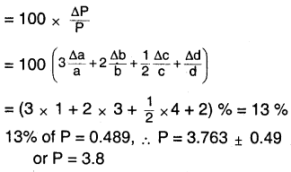Question 14.
A book with many printing errors contains four different formulas for the displacement y of a particle under going a certain periodic motion :
(a) y = a sin 2πt/T
(b) y = a sin vt
(c) y = (a/T) sin t/a
(d) y = $$(a\sqrt{2})$$ (sin 2πt/T + cos 2πt/T)
(a = maximum displacement of the particle, b a speed of the particle. T = time-period of motion). Rule out the wrong formulas on dimensional grounds.
Here we need the dimension of the LHS = dimension of RHL = L1
Also, the dimension of the argument of the trigonometric function = dimensionless

(a) Dimension of the argument of the sine function
$$\frac{T^{1}}{T^{1}}\mathrm{T}^{0}$$ = dimensionless.
Dimension of a = L1 = dimensions of y.
∴ This formula is dimensionally correct.

(b) Dimension of the argument of the sine function
= [LT-1] [T1] = L1
∴ This formula is dimensionally incorrect.

(c) Dimension of the argument of the sine function
= t/a = $$\frac{T}{L}$$ = [L-1] [T1] Also,
dimension of ($$\frac{a}{t}$$) = $$\frac{L}{T}$$ = LT-1 Clearly,
∴ This formula is dimensionally incorrect.

(d) Dimension of $$a\sqrt{2}$$ = L1 = dimension of y Dimension of the argument of the sine function
= dimension of the argument of the cosine function = 2π$$\frac{t}{T}$$ = $$\frac{T^{1}}{T^{1}}$$ = T° = dimensionless.
∴ This formula is dimensionally correct.

Question 15.
A famous relation in physics relates ‘moving mass’ m to the ‘rest mass’ m0 of a particle In terms of its speed and the speed of light, c. (This relation first arose as a consequence of special relativity due to Albert Einstein). A boy recalls the relation almost correctly but forgets where to put the constant
c. He writes :m = $$\frac{m_{0}}{\left(1-v^{2}\right)^{1 / 2}}$$ Guess where to put the missing c.
We need the dimension of the RHS to be equal to M1. Since m0 has the dimension of M1, the denominator must be dimensionless.

⇒ $$\left(1-v^{2}\right)^{\frac{y}{2}}$$ must be corrected to be dimensionless.
1 is dimensionless, v² has the dimensions [L1T-1]2 = L2 T-2

Using c, we need to make 1 – v² dimensionless. Since c has the same dimensions as v, c/v and v/c are both dimensionless.
∴ The corrected formula must be
m = $$\frac{m_{0}}{\left(1-v^{2} / c^{2}\right)^{1 / 2}}$$ or m = $$\frac{m_{0}}{\left(1-c^{2} / v^{2}\right)^{1/2}}$$

Since m0 is the rest mass, m = m0 when v = o. (body at rest)
For the first formula, when v = 0, m = undefined, which is wrong.
∴ Corrected formula is m = $$\frac{m_{0}}{\left(1-v^{2} / c^{2}\right)^{1/ 2}}$$

Question 16.
The unit of length convenient on the atomic scale is known as an angstrom and is denoted by Å: 1 Å = 10-10 m. The size of a hydrogen atom is about 0.5 Å. What is the total atomic volume in m3 of a mole of hydrogen atoms?
Radius of a hydrogen atom = r = 0.5 Å = 0.5 × 10-10m
Volume of a mole of hydrogen atoms = Volume of a hydrogen atom × Number of hydrogen atoms in 1 mole
= $$\frac{4}{3}$$πr3 × NA
= $$\frac{4}{3}$$ × 3.14 × ( 0.5 × 10-10)3 × 6.022 × 1023
= 3 × 10-7 m3 (rounded off to 1 significant figure)

Question 17.
One mole of an ideal gas at standard temperature and pressure occupies 22.4 L (molar volume). What is the ratio of molar volume to the atomic volume of a mole of hydrogen? (Take the size of hydrogen molecule to be about 1

1. Why is this ratio so large?
Atomic volume of a mole of hydrogen
= $$\frac{4}{3} \pi r^{3} \times N_{A}$$
= $$\frac{4}{3}$$ × 3.14 × (1 × 10-10)3 × 6.022 × 1023
= 2.52 × 10-6 m3
Required ratio
= $$\frac{22.4 L}{2.52 \times 10^{-6} m^{3}}$$
= $$\frac{22.4 \times 10^{-3}}{2.52 \times 10^{-6}}$$
= 9 × 103
This ratio is large because the intermolecular separation in a gas is much larger than the size of a molecule.

Question 18.
Explain this common observation clearly: If you look out of the window of a fast-moving train, the nearby trees, houses, etc. seem to move rapidly in a direction opposite to the train’s motion, but the distant objects (hilltops, the Moon, the stars, etc.) seem to be stationary. (In fact, since you are aware that you are moving, these distant objects seem to move with you).
Objects nearer to the eye subtend a greater angle in the eye than the distant objects. When we move, the change in this angle is less for distant objects than for near objects. So the distant objects seem stationary but nearer objects seem to move in the opposite direction.

Question 19.
The principle of ‘parallax’ is used in the determination of distances of very distant stars. The baseline AB is the line joining the Earth’s two locations six months apart in its orbit around the Sun. That is, the baseline is about the diameter of the Earth’s orbit. 3 × 1011m. However, even the nearest stars are so distant that with such a long baseline, they show parallax only of the order of 111 (second) of arc or so. A parsec is a convenient unit of length on the astronomical scale. It is the distance of an object that will show a parallax of 111 (second) of arc from opposite ends of a baseline equal to the distance from the Earth to the Sun. How much is a parsec in terms of metres?
Distance of the earth from the Sun =
= b = 1/2 × diameter of earth’s orbit
⇒ b= 0.5 × 3 × 1011 m
b = 1.5 × 1011 m
1 parsec = D = b/θ
= $$\frac{1.5 \times 10^{11} \mathrm{m}}{1^{11}}$$ = $$\frac{1.5 \times 10^{11}}{\left(\frac{1}{3600}\right)}$$
= $$\frac{1.5 \times 10^{11}}{\frac{1}{3600} \times \frac{\pi}{180}}$$ = 3 × 1016 m

Question 20.
The nearest star to our solar system is 4.29 light-years away. How much is this distance In terms of parsecs? How much parallax would this star (named Alpha Centaurl) show when viewed from two locations of the Earth six months apart in Its orbit around the Sun?
Distance of Alpha Centauri from the Earth = 4.29 ly
= 4.29 × 365 × 24 v 3600 × 3 × 108 = 4.06 × 1016m
1 parsec = 3.084 × 1016 m
∴ Distance = $$\frac{4.06 \times 10^{16}}{3.084 \times 10^{16}}$$ = 1.32parsec.
D = b/θ
b = 3 × 104, D = 4.06 × 1016 m
Parallax θ = 2 × D = 2.64

Question 21.
The Sun is a hot plasma (ionized matter) with its Inner core at a temperature exceeding 107 K, and its outer surface at a temperature of about 6000 K. At these high temperatures, no substance remains in a solid or liquid phase. In what range do you expect the mass density of the Sun to be, In the range of densities of solids and liquids or gases? Check if your guess is correct from the following data: mass of the Sun = 2.0 × 1030 kg, radius of the Sun = 7.0 × 108m.
Actual Density of the Sun = $$\frac{\text { Mass }}{\text { Volume }}$$
= $$\frac{2 \times 10^{30} \mathrm{kg}}{\frac{4}{3} \pi \times\left(7 \times 10^{8}\right)^{3} \mathrm{m}^{3}}$$
= 1.4 × 103 kg m-3

Question 22.
When the planet Jupiter is at a distance of 824.7 million kilometers from the Earth, Its angular diameter is measured to be 35.72″ of arc. Calculate the diameter of Jupiter.
D = 824.7 million km
θ = 35.7211
b = Dθ
=> b = 824.7 v 106 km × 35.7211
824.7 × 106 km × $$\frac{35.72}{3600}$$ × $$\frac{\pi}{180}$$ km
=1.429 × 105 km

### 1st PUC Physics Units and Measurements Additional Exercises Questions and Answers

Question 23.
A man walking briskly In the rain with speed v must slant his umbrella forward making an angle θ with the vertical. A student derives the following relation between θ and v: tan θ = v, and checks that the relation has a correct limit: as v -> o, θ -&gt; o, as expected. (We are assuming there is no strong wind and that the rain falls vertically for a stationary man). Do you think this relation can be correct? If not, guess the correct relation.
Since tan θ is dimensionless, we need the RHS of the equation tan θ = v to be dimensionless.
Let the speed of the rainfall be v1. Then, by the method of dimensions, we get that tan θ = v/v1 or v1/v
When the man is stationary (v = 0), he must hold the umbrella straight.
i.e θ = 0°
tan θ = 0 when v = 0
∴ The required relation is tan θ = v/v1Question 24.
It is claimed that two cesium clocks if allowed to run for 100 years, free from any disturbance, may differ by only about 0.02 s. What does this imply for the accuracy of the standard cesium clock in measuring a time – interval of 1 s?
Since the maximum error possible for 2 clocks is 0.02 s, the error for 1 clock = 0.01 s.
∴ Accuracy = $$\frac{3600 \times 24 \times 365 \times 100}{0.01}$$
= 3.15 × 1011
= 1 part in to11 to 1012

Question 25.
Estimate the average mass density of a sodium atom assuming its size to be about 2.5 Å. (Use the known values of Avogadro’s number and the atomic mass of sodium). Compare it with the density of sodium in its crystalline phase: 970 kg m-3. Are the two densities of the same order of magnitude? If so, why?
Mass of 1 mole (NA) of sodium atoms = 23 g.
Mass of 1 atom of sodium = $$\frac{23 \mathrm{g}}{\mathrm{N}_{\mathrm{A}}}$$
= $$\frac{23 g \times 10^{-3} k g}{6.022 \times 10^{23}}$$
= 3.819 × 10-26
Density of sodium atom = $$\frac{\text { Mass }}{\text { Volume }}$$
= $$\frac{3.819 \times 10^{-26}}{\frac{4}{3} \pi \times\left(2.5 \times 10^{-10}\right)^{3}}$$
= 583.5 kg m-3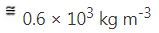970 kg m-3 and 0.6 × 103 kg m-3
are of the same order of magnitude because atoms are tightly packed in solid-state, so the atomic mass density is quite close to the density of the solid.

Question 26.
The unit of length convenient on the nuclear scale is a fermi: 1 f = 10-15m. Nuclear sizes obey roughly the following empirical relation:
r = r0A1/3
where r is the radius of the nucleus, A its mass number, and r0 is a constant equal to about 1.2 fm. Show that the rule implies that nuclear mass density is nearly constant for different nuclei. Estimate the mass density of sodium nucleus. Compare it with the average mass density of a sodium atom obtained in Exercise. 2.27.
Mass of 1 mole (NA) of atoms of an element = A g
= A × 10-3 kg
Mass of  1 atom = $$\frac{A \times 10^{-3} k g}{N_{A}}$$
Density of the atom = $$\frac{\text { Mass }}{\text { Volume }}$$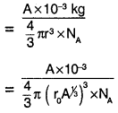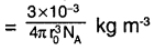which is a constant.
Mass density of the sodium nucleus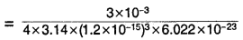= 2.30 × 1017 kg m -3
= 0.2 × 1018 kg m -3
$$\frac{\text { Nuclear density }}{\text { Atomic density }}$$ for sodium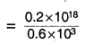= 0.33 × 1015
∴ Nuclear density is roughly 1015 to 1015 times the atomic density.

Question 27.
A LASER is a source of very intense, monochromatic, and unidirectional beam of light. These properties of laser light can be exploited to measure long distances. The distance of the Moon from the Earth has been already determined very precisely using a laser as a source of light. A laser light beamed at the Moon takes 2.56 s to return after reflection at the Moon’s surface. How much Is the radius of the lunar orbit around the Earth?
Time for the laser reach the moon
= 1/2 × 2.56 s = 1.28 s
∴ Distance from the Earth to the moon = 1.28 s × speed of light in vacuum = 1.28 × 3 × 108ms-1
= 3.84 × 108m
∴ The radius of the lunar orbit = 3.84 × 108m.

Question 28.
A SONAR (sound navigation and ranging) uses ultrasonic waves to detect and locate objects underwater. In a submarine equipped with a SONAR the time delay between generation of a probe wave and the reception of its echo after reflection from
an enemy submarine is found to be 77.0 s. What is the distance of the enemy submarine? (Speed of sound in water =
1450 ms-1).
Time for the SONAR to reach the enemy submarine = 1/2 × 77.0 s = 38.5s
Distance of the enemy submarine = 38.5 s × speed of Sound in water
= 38.5 s × 1450 m s-1 = 55825 m = 55.8 km.

question 29.
The farthest objects in our Universe discovered by modern astronomers are so distant that light emitted by them takes billions of years to reach the Earth. These objects (known as quasars) have many puzzling features, which have not yet been satisfactorily explained. What is the distance in km of a quasar from which light takes 3.0 billion years to reach us?
Distance of the quasar from the earth = Distance travelled by light in 3 billion years
= 3 × 105 kms-1 × 3 × 109 × 365 × 24 × 3600
= 2.8 × 1022 km.Question 30.
It is a well-known fact that during a total solar eclipse-the disk of the moon almost completely covers the disk of the Sun. From this fact .determine the approximate diameter of the moon.
AC = AD, AB = AE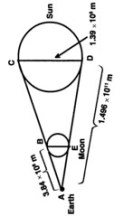From the data, we get the distances of the Sun and Moon from the Earth, as well as the diameter of the Sun.
Using the concept of similar triangles
ΔABE ~ ΔACD ⇒ $$\frac{A B}{A D}=\frac{B E}{C D}$$
⇒ BE = diameter of the moon
= CD × $$\frac{\mathrm{AB}}{\mathrm{AD}}$$ =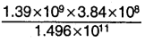= 3568 km.

Question 31.
A great physicist of this century (P.A.M. Dirac) loved playing with numerical values of Fundamental constants of nature. This led him to an interesting observation. Dirac found that from the basic constants of atomic physics (c, e, mass of electron, mass of proton) and the gravitational constant G, he could arrive at a number with the dimension of time. Further, it was a very large number, its magnitude being close to the present estimate on the age of the universe (~15 billion years). From the table of fundamental constants in this book, try to see if you too can construct this number (or any other Interesting number you can think of). If its coincidence with the age of the universe were significant, what would this imply for the constancy of fundamental constants?
[c] = [LT-1]
[e] = [IT]
[me] = [mp] = [M]
[G] = [M-1 L3 T2]
[∈o]= [M-1 L-3 T4 I2]
By the given condition
[c]a [e]b [me]c[mp]d [G]e [∈o]f = [T]
[LT-1]a [IT]b [M]c[M]d [M-1L3T-2]e × [M-1 L-3 T4I2]f = [T]
[Mc+d-e-f La+3e-3f T-a+b-2e+4f Ib+2f]
[M0 L0 T1 I0]

Comparing the powers of M, L, T and I, we get
⇒c + d-e – f               = 0    →(1)
a + 3e – 3f                  = 0    →(2)
-a + b -2e + 4f           = 1    →(3)
b + 2f                         = 0    →(4)
We have 4 equations but 6 unknowns. Hence we cannot arrive at a unique solution. But we can get the solutions by guessing the values for some of the unknowns.
Let a = -3, e = -1, f = -2 c = -2, Now we have d = -1 and b = 4
∴ The equation is of the form =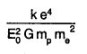where k is a constant.

### 1st PUC Physics Units and Measurements One Mark Questions and Answers

Question 1.
What is a unit?
The standard quantity in terms of which a physical quantity is measured is called the unit of that physical quantity.

Question 2.
What are the fundamental quantities?
The quantities, which are independent of other physical quantities are called fundamental quantities.

Question 3.
What are derived quantities?
The quantities, which are derived from fundamental quantities are called derived quantities.

Question 4.
What is dimensional formula?
A formula in which a physical quantity is expressed in terms of fundamental quantities is called the dimensional formula.Question 5.
What are the dimensions?
Dimensions of a physical quantity are the powers to which the fundamental quantities must be raised to obtain the unit of a given physical quantity.

Question 6.
State the principle of homogeneity of dimensions.
The principle of homogeneity of dimensions states that the dimensional formula of all the terms in an equation must be the same.

Question 7.
Write the dimensional formula for velocity.
LT-1.

Question 8.
Write the dimensional formula for force.
MLT2.

Question 9.
Write the dimensional formula for power.
ML2T3.

Question 10.
Name a physical quantity that has a unit but no dimension.
Angle. (Bangalore South, North 05)

Question 11.
Write the dimensions of the coefficient of viscosity?
[η] = [M1L-1T-1].
∴ Dimensions of co-efficient of viscosity are 1 in mass, -1 in length and -1 in time.

Question 12.
Name the SI unit of weight.
SI unit of weight is Newton (N).Question 13.
Write the number of significant figures in each of the following measurements

1. 1.67 × 10-27 kg
2. 0.270 cm

1. Three significant figures.
2. Three significant figures.

Question 14.
Name the S.I unit used to express the amount of substance.
Mole.

Question 15.
How many newtons make 1 kg wt?
9.8 N = 1 kg wt.

### 1st PUC Physics Units and Measurements Two Mark Questions and Answers

Question 1.
Name the fundamental quantities.
Mass, length, time, temperature, amount of substance, luminous intensity and electric current.

Question 2.
What are derived quantities? Give examples.
The quantities, which are derived from fundamental quantities, are called derived quantities. E.g: Velocity is derived from length and time, Charge is derived from current and time. Area is derived from length, Volume is derived from the length, etc.

Question 3.
Mention the applications of dimensional equations.
Dimensional equations are used :

1. To check the correctness of an equation.
2. To derive the relation between different physical quantities.
3. To convert one system of units into another system.Question 4.
Using the method of dimension check the correctness of the equation, v2 = u2+2as
v2 = u2+2as, writing the dimensional formula for each of them
[LT-1]2 = [LT-1]2 + [LT-2] [L]
[IL2T-2] = [L2 T-2] + [L2 T-2]
All the terms have the same dimensional formula & hence the Equation is dimensionally correct.

Question 5.
Find the area of the circle of radius 3.458 cm up to correct significant figures.
A = πr²
= 3.142 × 3.4582 = 37.57 cm2

Question 6.
The radius of a solid sphere is measured to be 11.24 cm. What is the surface area of the sphere to appropriate significant figures?
r = 11.24 cm
Surface area = 4 πr² = 4 × 3.142 × (11.24)2
= 1588 cm2

Question 7.
If A = (12.0 ±0.1) cm and
B = (8.5 ± 0.5) cm, find:
(i) A + B and (ii) A – B
(i) A + B = (12.0 ± 0.1) + (8.5 ± 0.5)
= (20.5 ±0.6) cm
(ii) A-B = (12.0 ± 0.1) – (8.5 ±0.5)
= (3.5 ± 0.6) cm.Question 8.
Magnitude of force F experienced by a certain object moving with speed V is given by F = Kv2, where K is a constant. Find the dimensions of K.
F =Kv2
Taking dimensions of each term, we get
[M L T-2] = [ K ] [L T-1]2
⇒ [K] = $$\frac{\left[M L T^{-2}\right]}{\left[L^{2} T^{-2}\right]}$$
[K] = [ML-1]

Question 9.
For the determination of ‘g’ using a simple pendulum, measurenents of I and T are required. Error in the measurement of which of these will have a larger effect on the value of ‘g’ thus obtained and why? What is done to minimise this error?
The formula for ‘g’ is
g = 4 π² $$\frac{I}{\mathrm{T}^{2}}$$
∴ $$\frac{\Delta g}{g}=\frac{\Delta I}{I}+2 \frac{\Delta T}{T}$$
Error in the measurement of T will have a larger effect on the obtained value of ‘g’. To minimise this error, a large number of measurements of T is taken.

### 1st PUC Physics Units and Measurements FourFive Mark Questions and Answers

Question 1.
The side (r) of a cube is measured as (11.3 ± 0.1) cm. What is the volume of the cube?
Volume V = r3
$$\frac{\Delta r}{r}$$ = $$\frac{0.1}{11.3}$$ = 0.009
$$\frac{\Delta V}{V}$$= 3 × $$\frac{\Delta r}{r}$$ = 0.027
V = (11.3)3 = 1442.9 cm3
ΔV = 1442.9 × 0.027 = 38.96 cm3
Volume of the cube = (1442.9 ± 38.96)cm3

Question 2.
A drop of olive oil of radius 1 mm spreads into a circular film of diameter 40 cm on a water surface. Estimate the size of an oil molecule.
Volume of the oil is conserved. Radius of oil drop = R = 1 mm
Radius of the oil film on the water surface is r = 20 cm
The thickness of the oil film formed represents the size of the oil molecule.
$$\frac{4}{3} \pi \mathrm{R}^{3}$$ = πr² × size of a molecule
⇒Size of a molecule = $$\frac{4}{3} \times \frac{R^{3}}{r^{2}}$$
= $$\frac{4}{3} \times \frac{\left(1 \times 10^{-3}\right)^{3}}{\left(20 \times 10^{-2}\right)^{2}}$$
= 0.33 × 10-7 m

Question 3.
A large fluid star oscillates in shape under the influence of its own gravitational field. Using dimensional analysis, find the expression for period of oscillation (T) in terms of radius of the star (R), mean density of the Fluid (p) and universal gravitational constant (G).
T α Ra $$\rho$$b Gc
Considering the dimensions of the terms,
[M0 L0 T1] = [L1]a [ML-3]b [M-1 L3 T-2]c
[M0 L0 T1] = [Mb-c La-3b+3c T-2c]
Equating the powers of M, L and T,
we get b – c = 0
a – 3b + 3c = 0
– 2c = 1
⇒b = c, a = 0,c = -1/2
∴ T = K R° $$\rho^{-x / 2} G^{-y / 2}$$ = $$\frac{\mathrm{K}}{\sqrt{\rho \mathrm{G}}}$$
where K is a constant.

Question 4.
The length and breadth of a rectangle are (5.7 ±0.1) cm and (3.4 ±0.2) cm. Calculate the area of the rectangle with error limits.
I = (5.7 ± 0.1) cm
b = (3.4 ± 0.2) cm
Area = lb = 5.7 × 3.4 = 19.38 cm2
$$\frac{\Delta A}{A}=\frac{\Delta I}{I}+\frac{\Delta b}{b}$$
⇒ ΔA = $$\left(\frac{0.1}{5.7}+\frac{0.2}{3.4}\right)$$ × 19.4
= 1.5 cm2
∴ A = (19.4 ± 1.5) cm2

Question 5.
From the bottom of a rock mountain, If the angle of elevation of its top increases from 30° to 45° on moving 100 m towards the rock In the horizontal direction through the base of the rock.
∆ In ABC, $$\frac{h}{x}$$ = tan 45°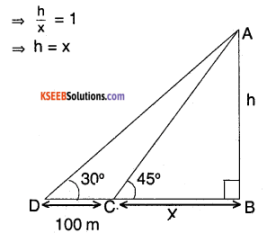In ABD, $$\frac{\mathrm{h}}{\mathrm{x}+100}$$
= tan 30°
⇒ $$\frac{h}{h+100}=\frac{1}{\sqrt{3}}$$
⇒ $$\mathrm{h}=\frac{100}{(\sqrt{3}-1)}$$
h = 136.6 m

Question 6.
If the units of force, velocity and energy are 100 dyne, 10cm/sec and 400 ergs respectively, what will be the units of mass, length and time?
F = 100 dyne = [M LT-2]
V = 10 cm s-1 = [LT-1]
E = 400 ergs = [M L2T-2]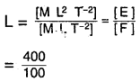L = 4 cm
[L T-1] = 10
⇒4T-1 = 10
⇒T = 0.4 sec
[M L T-2] = 100
⇒M 4 × (0.4)-2 = 100
⇒M = 4 gm

Question 7.
If the displacement of a body, = (200 ± 5) m and time taken by it is t = (20 ± 0.2) s, find the percentage error In the calculation of velocity.
$$\frac{\Delta V}{V} \times 100 \%=\frac{\Delta S}{S} \times 100 \%+\frac{\Delta t}{t} \times 100 \%$$
= $$\frac{5}{200}$$ × 100+$$\frac{0.2}{20}$$ × 100
= 2.5 + 1
= 3.5%

Question 8.
Using the principle of homogeneity of dimensions, find which of the following is correct.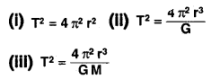Where T is the time period, G Is the gravitational constant, M is the mass and r is the radius of the orbit.
Dimensions of LHS in each case =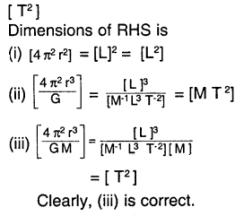Question 9.
A physical quantity Q is given by Q = $$\frac{A^{2} \cdot B^{3 / 2}}{C^{4} \cdot D^{/ 2}}$$. The percentage error In A, B, C and D are 1%, 2%, 4% and 2% respectively. Find the percentage error in Q.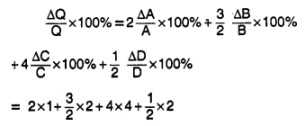= 22%

### 1st PUC Physics Units and Measurements Questions from the previous question papers

Question 1.
Check the correctness of the following equation.
1. v = u + at
2. s = ut + 1/2 at2
1. Given equation is v = u + at , Writing the dimensional formula of each of them we get,
[LT-1] = [LT-1] + [LT2][T]
[LT-1] = [LT-1] + [LT-1]
All the terms have the same dimensional formula and hence the equation is dimensionally correct.

2. Given equation is s = ut + 1/2 at2
Writing the dimensional formula of each of them we get,
[L] = [LT-1][T] + [LT-2][T2]
[L] = [L]+[L]
All the terms have the same dimensional formula and hence the equation is dimensionally correct.Question 2.
The period of oscillation (T) of a simple pendulum Is found to depend on
i) mass of the pendulum (m)
ii) length of the pendulum (I)
iii) acceleration due to gravity (g).
Derive the relation between them.
Let T ∝mx Iy gz
T = k mx Iy gz ……………….. (1)
where ‘k’ is constant of proportionality. Writing the dimensional formula of equation (1),
[T] = [M]x[L]y[LT-2]z
[M0 L0 T] = [MxLy+zT-2z]
Comparing the dimensions we get,
x = 0, y + z = 0, -2z = 1
x = 0, y = – z , z = -1/2
x = 0, y = 1/2 , z = -1/2
Substituting in (1) we get,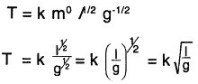Experimetnally the value of constant ‘k’ is found to be 2π.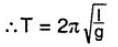Question 3.
Convert MKS unit of force Into the CGS unit. Or Relate newton and dyne. OR Show that 1 newton =105 dyne by method of dimension.
Let MKS unit of force = n × CGS unit of force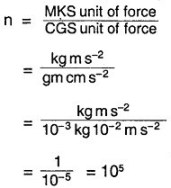∴ MKS unit of force = 105 CGS unit of force i.e., 1 newton = 105

Question 4.
Mention the limitations of dimensional analysis.
The limitations of dimensional equations are:

• Even though a given equation is dimensionally correct, it is not possible to conclude that it is truly correct.
• The constant of proportionality cannot be determined.
• This method cannot be used for deriving equations involving trigonometric and logarithm functions.
• This method cannot be used to derive equations where addition or subtraction is involved.

Question 5.
Check the correctness of the equation sn = u+$$\frac{a}{2}$$ (2n-1) by dimensional analysis, where the symbols have the usual meaning.
Writing the dimensional formula of each of them, we get
L1 = LT-1+LT-2[T]
L1 = LT-1+LT-1
Since all the terms do not have the same dimensional formula, the equation is dimensionally not correct.

Question 6.
Check the correctness of equation, E = mc2 by dimensional analysis, where the symbols have their usual significance.
Writing the dimensional formula of each of them,
we get M1 L2T-2 = M1 L2T-2
All the terms have the same dimensional formula & hence the equation is dimensionally correct.Question 7.
Define dimensions of a physical quantity. Write the dimensions of velocity, force, and energy.
Dimensions of physical quantities are the powers to which the fundamental quantities, must be raised to obtain that physical quantity.
1) [velocity] = [M0L1T-1]
Dimension of velocity in mass 0, in length 1, & in time -1.
2) [Force] = [M1L1T-2]
Dimension of force in mass 1, in length 1 & in time -2.
3) [Energy] = [M1L2T-2]
Dimension of energy in mass 1, in length 2 & in time -2.

Question 8.
Show that 1 Joule = 107erg by the method of dimension.
Let MKS unit of work
=n × CGS unit of work.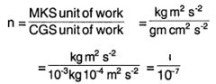∴ MKS unit of work = 107 CGS unit of work.
i.e 1 J = 107 erg.

Question 9.
Write a note on the SI system.
SI system represents the “International system of units”. It was adopted in 1971 at the general conference on Weights and Measures :
S.I. system has seven fundamental units. They are

1. meter for length,
2. kilogram for mass
3. second for time
4. ampere for electric current
5. Kelvin for temperature
6. candela for luminous intensity
7. mole for Amount of substance.

There are 2 supplementary units, They are

1. radian for measurement of angle in a plane.
2. steradian for measurement of angle in solid angle.

Question 10.
Write any two applications and any two limitations of dimensional analysis.
Dimensional equations are used :

• To check the correctness of an equation.
• To derive the relation between different physical quantities.
• To convert one system of units into another system.

The limitations of dimensional equations are:

• Even though a given equation is dimensionally correct, it is not possible to conclude that it is truly correct.
• The constant of proportionality cannot be determined.
• This method cannot be used for deriving equations involving trigonometric and logarithm functions.
• This method cannot be used to derive equations where addition or subtraction is involved.

Question 11.
Write the dimensions for
i) force
ii) pressure
iii) volume and
iv) velocity.